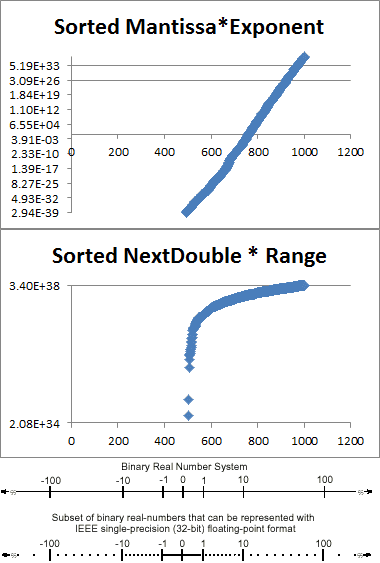# Best way to generate a random float in C# [closed]

Best approach, no crazed values, distributed with respect to the representable intervals on the floating-point number line (removed “uniform” as with respect to a continuous number line it is decidedly non-uniform):

``````static float NextFloat(Random random)
{
double mantissa = (random.NextDouble() * 2.0) - 1.0;
// choose -149 instead of -126 to also generate subnormal floats (*)
double exponent = Math.Pow(2.0, random.Next(-126, 128));
return (float)(mantissa * exponent);
}
``````

(*) … check here for subnormal floats

Warning: generates positive infinity as well! Choose exponent of 127 to be on the safe side.

Another approach which will give you some crazed values (uniform distribution of bit patterns), potentially useful for fuzzing:

``````static float NextFloat(Random random)
{
var buffer = new byte;
random.NextBytes(buffer);
return BitConverter.ToSingle(buffer,0);
}
``````

An improvement over the previous version is this one, which does not create “crazed” values (neither infinities nor NaN) and is still fast (also distributed with respect to the representable intervals on the floating-point number line):

``````public static float Generate(Random prng)
{
var sign = prng.Next(2);
var exponent = prng.Next((1 << 8) - 1); // do not generate 0xFF (infinities and NaN)
var mantissa = prng.Next(1 << 23);

var bits = (sign << 31) + (exponent << 23) + mantissa;
return IntBitsToFloat(bits);
}

private static float IntBitsToFloat(int bits)
{
unsafe
{
return *(float*) &bits;
}
}
``````

Least useful approach:

``````static float NextFloat(Random random)
{
// Not a uniform distribution w.r.t. the binary floating-point number line
// which makes sense given that NextDouble is uniform from 0.0 to 1.0.
// Uniform w.r.t. a continuous number line.
//
// The range produced by this method is 6.8e38.
//
// Therefore if NextDouble produces values in the range of 0.0 to 0.1
// 10% of the time, we will only produce numbers less than 1e38 about
// 10% of the time, which does not make sense.
var result = (random.NextDouble()
* (Single.MaxValue - (double)Single.MinValue))
+ Single.MinValue;
return (float)result;
}
``````

Floating point number line from: Intel Architecture Software Developer’s Manual Volume 1: Basic Architecture. The Y-axis is logarithmic (base-2) because consecutive binary floating point numbers do not differ linearly.